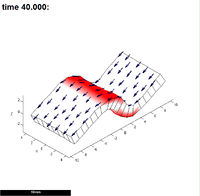Step size (dt) is 10. Is the 'S' shape correct? Simple mesh of 382 elements equally spaced vertices. Superimposed are the polarity arrows (pointing bottom left) and purple and blue factors that control local growth rates.Step size (dt) is 1. Is the 'S' shape correct? It is different so we reduce it again...Step size (dt) is 0.1. This shape is very similar to the previous one so we might conclude that it is not necessary to make the stepsize this short, a stepsize of 1 is OK.